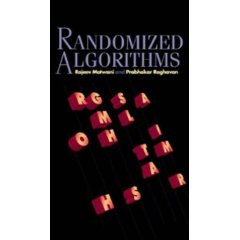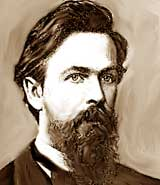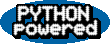# Doing the Martin Shuffle (with your iPod)

I've got an iPod shuffle. A lot of people do. So you probably know they're great, but they don't have a display. If a song is playing and you want to know what it is, you're out of luck. I can think of two ways that could be fixed:

1. A button sequence could mark the current song; when you later connect your iPod to a computer, iTunes could display the marked songs. You don't get immediate gratification, but you do eventually find out the name of the song.

2. iTunes could be modified to use the Apple text-to-speech module to speak the song's metadata (e.g. title and artist) and store it as an MP3 or AAC on the iPod. You press some other button sequence to hear the metadata. If you want, you can set an option in iTunes to speak the metadata before every song. Of course you can choose the voice you want to use, etc.

I believe that both could be implemented with just a software update, without altering the iPod hardware. You don't need a new button, you can use a sequence of existing ones, such as double-click on the 'play' button. Or the rarely-used battery check button. Note if you use these button sequences on an iPod that hasn't been updated, there are no ill effects. So go ahead, Steve; I grant you rights to these ideas, free of charge.Ipod Shuffle

## The Martin Shuffle

The two ideas above are good if you want to identify a song, but not if you want to find a song. But for that my friend Charles Martin came up with an idea that works with no hardware or software changes needed. I call it the Martin Shuffle, and it works like this:

• In iTunes, sort your playlist by song title or artist, whichever you think you will want to search for.
• Now on your iPod, suppose you want to find something. To make it concrete, suppose you want to find Something. First you listen to the current song long enough to identify it. If it is alphabeticly close (say, Someone to Watch Over Me or Summertime) you press the 'next' or 'previous' song button in sequential (non-shuffle) mode until you arrive at your target. If the current song is far away (say, Funkytown) you go into shuffle mode and hit the 'next' button (thereby randomly jumping to another song) until you do get close; then switch to non-shuffle mode.

Note this is a randomized algorithm; you use randomness to solve a deterministic problem faster than you could without randomness.

So now there are two questions: how close do you have to get before you switch to non-shuffle mode, and how long will it take, on average, to find a song with this approach?Charles MartinRandomized Algorithms

## Markov Decision Processes to the Rescue

What we need is a policy for when to hit the shuffle button and when to switch to the sequential button. The tricky part is that shuffling is random -- can we determine the optimal policy when we don't know where we'll end up? It turns out that we can if we treat this as a Markov Decision Process, or MDP. In an MDP you need to define the following:

• States. For the iPod, the state is just the current song, so if there are N songs, there are N states. For a 1GB iPod Shuffle, assume N = 250.
• Actions. We define two actions: Shuffle and Sequential. We define the sequential action as moving all the way to the target (rather than moving just one position towards the target); it is a single action consisting of multiple button presses.
• Transitions. For each (action, state) pair, we enumerate the possible states that the model might transition to, each with a probability. For Sequential we always transition to the target. For Shuffle we transition to each of the other states with equal probability.
• Costs. For each transition there is an assoicated cost. We'll measure the cost in seconds, and assume that Sequential costs 1 second per button press. Shuffle takes somewhat longer, because you have to stop to identify the song and remember where it is in alphabetical order. Let's call it T seconds, and consider values of T from 1 to 10.

Now the basic idea for finding the optimal policy in an MDP is simple:

For each state of the problem, choose the action that minimizes the sum of the cost of the action and the expected cost of getting from the resulting state to the target.
We will follow tradition and use the notation V[s], where V stands for value, to denote the cost of a state, but these really are costs: low numbers are better. Once we solve an equation for V[s] we can easily determine the optimal policy.

First assume that the target song is number N/2. (We can do this without loss of generality because the songs are actually arranged in a circle, not a line segment: from the last song you can go forward to the first. So the numbering is arbitrary because every point on a circle is isomorphic.) Then the cost of a state is the minimum of the cost of sequentially moving to the target (which is the absolute value of the distance to the target, |s-t|) and the cost of shuffling and then finding the way to the target (which is T plus the average cost of wherever we end up by shuffling):

V[s] = min(|s-t|, T + (1/N) Σr V[r]

## The Value Iteration Algorithm

We can't directly solve this equation because V appears on both left and right hand sides: the value of a state is defined in terms of the values of other states. So how do we break the loop? It turns out the equation can be solved by an algorithm called value iteration that starts with an initial guess for all V[s] and then updates the guesses repeatedly, until there are no more changes (or until all changes are smaller than epsilon). This iterative algorithm is guaranteed to converge.

To initialize the estimates of V for each state let's just assume you always use the Sequential strategy and thus each V[s] is the absolute value of s - t.

To update the value for a state, we check to see if we could do better by switching to the Shuffle strategy. The expected value of Shuffle is the cost T of shuffling and identifying the resulting song, plus the average value of the V[r] for each possible resulting state r (which I originally thought was every state except the current state, but an interesting article by Brian E. Hansen convinced me that it is possible to randomly skip from a song to the same song).A.A. Markov

## Coding a Solution

We can now show some code for valueiteration on the iPod problem. (You can also see code for a general MDP solver.)

 ```def valueiteration(N, T, epsilon=0.001): t = N/2 states = range(N) V1 = [abs(s-t) for s in states] V2 = [0.0 for s in states] while max([abs(V2[s]-V1[s]) for s in states]) > epsilon: shufflecost = T + avg([V1[r] for r in states]) for s in states: V2[s] = min(abs(s-t), shufflecost) V1, V2 = V2, V1 return V2 ```

This is Python code; if you're not familiar with Python you should know that [abs(s-t) for s in states] iterates s over each element of states and collects the values of abs(s-t) into a list. Also, range(N) returns a list of the numbers from 0 to N-1, inclusive, and V1, V2 = V2, V1 swaps V2 and V1. All assignment in Python is done by moving pointers, not by creating copies of objects. The rest you should be able to figure out.

Besides valueiteration, all we need is a trivial function to compute the average (mean) of a sequence of numbers, and a main function that calls valueiteration and prints out some statistics on the results:

 ```def avg(nums): return float(sum(nums)) / len(nums) def main(N=250, Ts=[1,5,10]): global V t = N/2 for T in Ts: V = valueiteration(N, T) print 'T=%d (N=%d) ==> shuffle when %d or more away' % ( T, N, (t - min([s for s in range(N) if V[s] == t-s]))) print 'Mean: %.1f, Median: %.1f; Max: %.1f' % ( avg(V), sorted(V)[N/2], max(V)) print ```Python

## And the Answer is...

Running main() produces these results:

 ```T=1 (N=250) ==> shuffle when 15 or more away Mean: 14.8, Median: 15.8; Max: 15.8 T=5 (N=250) ==> shuffle when 35 or more away Mean: 30.4, Median: 35.4; Max: 35.4 T=10 (N=250) ==> shuffle when 50 or more away Mean: 40.0, Median: 50.0; Max: 50.0 ```

So that answers our two questions. Assuming 5 seconds per shuffle, then on a 250 song iPod you should use the policy of shuffling until you get within 35 songs, and you should expect to spend 30.4 seconds on average finding a song. The switching point and the total time go down if you can identify a song faster, and up if you are slower.

On a 125 song (512MB) iPod, you should shuffle until you get within 25 songs, and expect to spend 20.0 seconds (assuming 5 seconds to shuffle), according to this output:

 ```T=1 (N=125) ==> shuffle when 11 or more away Mean: 10.2, Median: 11.2; Max: 11.2 T=5 (N=125) ==> shuffle when 25 or more away Mean: 20.0, Median: 25.0; Max: 25.0 T=10 (N=125) ==> shuffle when 35 or more away Mean: 25.4, Median: 31.0; Max: 35.4 ```

Happy shuffling!

OK, now that the iPod Nano (with a display) is out, some may claim that this page is irrelevant. But I think it is still of use for those with shuffles, or for those interested in MDPs.

A reader asked why I have global V in main. The reason is that that way I have the value of V available for inspection in the interactive interpreter, even though I don't return the value.

Andre Kloss presents a Python script to show the last played songs; you install the script in the root directory of your iPod and run it from there when you connect to your computer.

Andre also points out that Martin Fiedler's shuffle database generator can be used to change the order of shuffling; you may be able to choose an order that makes searching easier for you.

I coded the value iteration algorithm from memory without consulting a reference, and it turns out I forgot an important factor: the future discounting factor, gamma (γ). This can have an effect on convergence of the values, particularly for problems where there are paths that accumulate infinite value. This is not an issue for our iPod problem, but to verify that my results are reasonable, I wrote a function, run to run a simulated random search for a song, on an N song iPod, with shuffle time T, and with the policy of shuffling when farther than p songs away from the target. I also updated the main function to compare the simulation results to the calculated MDP results:

 ```def main(N=250, Ts=[1,5,10], Nruns=100000): global V t = N/2 for T in Ts: V = valueiteration(N, T) p = t - min([s for s in range(N) if V[s] == t-s]) p_from_sim = min(range(2,60), key=lambda p: avgruntime(N, T, p, Nruns)) print 'T=%d (N=%d) ==> shuffle when %d or more away (%d from simulation)' % ( T, N, p, p_from_sim) print 'Mean: %.1f (%.1f from simulations), Median: %.1f; Max: %.1f' % ( avg(V), avgruntime(N, T, p, Nruns), sorted(V)[N/2], max(V)) print import random def run(N, T, p): t = N/2 state = random.randrange(N) time = 0 while (state != t): if abs(state-t) < p: time += abs(state-t) state = t else: time += T state = random.randrange(N) return time def avgruntime(N, T, p, Nruns): return avg([run(N, T, p) for _ in range(Nruns)]) main(125) main(250) ```

Here are the new output results:

 ```T=1 (N=125) ==> shuffle when 11 or more away (12 from simulation) Mean: 10.2 (10.2 from simulations), Median: 11.2; Max: 11.2 T=5 (N=125) ==> shuffle when 25 or more away (26 from simulation) Mean: 20.0 (20.0 from simulations), Median: 25.0; Max: 25.0 T=10 (N=125) ==> shuffle when 35 or more away (35 from simulation) Mean: 25.4 (25.4 from simulations), Median: 31.0; Max: 35.4 T=1 (N=250) ==> shuffle when 15 or more away (17 from simulation) Mean: 14.8 (14.9 from simulations), Median: 15.8; Max: 15.8 T=5 (N=250) ==> shuffle when 35 or more away (35 from simulation) Mean: 30.4 (30.3 from simulations), Median: 35.4; Max: 35.4 T=10 (N=250) ==> shuffle when 50 or more away (50 from simulation) Mean: 40.0 (40.0 from simulations), Median: 50.0; Max: 50.0 ```

The average runtimes of the simulations are all within 0.1 of the values computed by my MDP routine. The best policy found by simulation is never more than 1 or 2 away from the MDP best policy. (It is not always the same because often there are multiple policies with very similar expected values.) Overall these simulations give me some confidence that my analysis and code is correct.

## Analytic Solution

Darius Bacon wrote in to say that "the best cutoff was 'obviously' going to be O(sqrt(N)) (for T = 1, anyway)", and that he had confirmed by checking my numbers that it was sqrt(N T). Darius may be more discerning than most people (including me) in seeing this as 'obvious,' but those who know the classic drop two eggs from a building puzzle may remember the answer was sqrt(N) there as well.

Later, Houman Alborzi wrote to say the same thing, and provided an analytic derivation:

```Let cost(i) indicate the average cost of finding a song that has
distance i from current song. The recurrence equations for cost(i) are
then:

cost(0) = 0
cost(i) = min(1+cost(i-1), T+P)
where P is average cost of a position resulting from shuffle:
for N an even number
P = 2/N(cost(0)+cost(1)+cost(2)+...+cost(N/2))
for N an odd number
P = 2/N(cost(0)+cost(1)+cost(2)+...+cost(N/2)+1/2(cost((N+1)/2))

The strategy is to shuffle when i is bigger than a threshold value t, that is:
for i<=t      :cost(i)=1+cost(i-1)=i
for i>=t+1 :cost(i)=T+P

for even and odd N:
N/2 * P = (0 + 1 + ... + t + (N/2-t)*(T+P)
N/2 * P =  (t+1)*t/2 + (N/2-t)*(T+P)
P*(N/2 -(N/2-t))=  (t+1)*t/2 + (N/2-t)*T
P*t =  (t+1)*t/2 + (N/2-t)*T
P=  (t+1)/2 + N/2*T/t-T
so:
for i<=t :cost(i)=i
for i>=t+1 :cost(i)=(t+1)/2 + N/2*T/t

Find the threshold value t that minimizes P, the average case cost of
the strategy:
dP/dt = 0
1/2 - N*T/2/t^2 = 0
or,
t=sqrt(N T) (neglecting the fact that t should be an integer)

Moreover, P can be calculated as:(again neglecting the fact that t
should be an integer)
P = (t+1)/2 + N/2*T/t-T
P = sqrt(N T)/2+1/2 + sqrt(N T)/2-T
P = sqrt(N T)+1/2 -T
P = t+1/2 -T
```iPod Nano

Peter Norvig